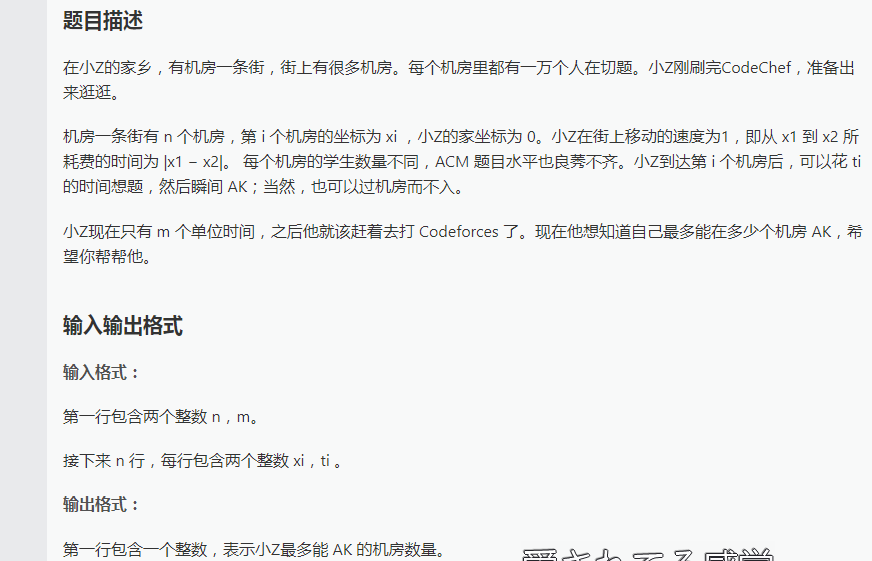# luogu P2107 小Z的AK计划1 #include<bits/stdc++.h>
2 #define ll long long
3 #define uint unsigned int
4 #define ull unsigned long long
5 using namespace std;
6 const int maxn = 110000;
7 struct shiki {
8     ll x, t;
9 }a[maxn];
10 ll heap[maxn << 1], tot = 0, num = 0;
11 ll n, m, top = 0;
12 ll ans = 0;
13
15     ll x = 0, y = 1;
16     char ch = getchar();
17     while(!isdigit(ch)) {
18         if(ch == '-') y = -1;
19         ch = getchar();
20     }
21     while(isdigit(ch)) {
22         x = (x << 1) + (x << 3) + ch - '0';
23         ch = getchar();
24     }
25     return x * y;
26 }
27
28 inline void up(int p) {
29     while(p > 1) {
30         if(heap[p] > heap[p / 2]) {
31             swap(heap[p], heap[p / 2]);
32             p /= 2;
33         }
34         else break;
35     }
36 }
37
38 inline void down(int p) {
39     int s = p * 2;
40     while(s <= tot) {
41         if(s < tot && heap[s] < heap[s + 1]) s++;
42         if(heap[p] < heap[s]) {
43             swap(heap[s], heap[p]);
44             p = s, s = p >> 1;
45         }
46         else break;
47     }
48 }
49
50 inline void extract() {
51     heap = heap[tot--];
52     down(1);
53 }
54
55 inline void insert(ll k) {
56     heap[++tot] = k;
57     up(tot);
58 }
59
60 inline bool cmp(shiki a, shiki b) {
61 return a.x < b.x;}
62
63 inline int get_top(){return heap;}
64
65 int main() {
67     for(int i = 1; i <= n; ++i) {
69         if(x <= m && t <= m) {
70             a[++top].x = x;
71             a[top].t = t;
72         }
73     }
74     sort(a + 1, a + top + 1, cmp);
75     for(int i = 1; i <= top; ++i) {
76         insert(a[i].t);
77         num += (a[i].x - a[i - 1].x) + a[i].t;
78         if(num <= m) ans++;
79         if(num > m) {
80             num -= get_top();
81             extract();
82         }
83     }
84     printf("%lld\n", ans);
85     return 0;
86 } 

posted @ 2018-10-26 18:32  YuWenjue  阅读(70)  评论(0编辑  收藏